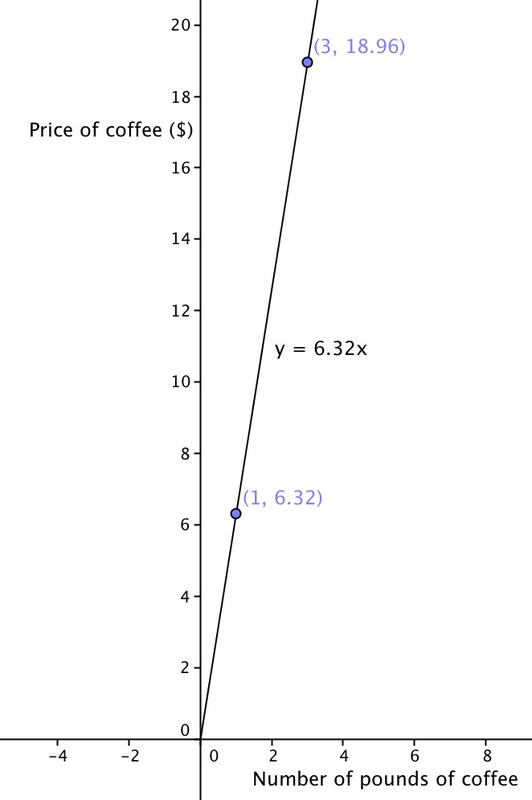# Coffee by the Pound

Alignments to Content Standards: 8.EE.B.5

Lena paid \$18.96 for 3 pounds of coffee. 1. What is the cost per pound for this coffee? 2. How many pounds of coffee could she buy for \$1.00?
3. Draw a graph in the coordinate plane of the relationship between the number of pounds of coffee and the total cost.
4. In this situation, what is the meaning of the slope of the line you drew in part (c)?

## IM Commentary

A slight modification to this problem would make this appropriate for the 7th grade level (see 7.RP.2.d Coffee by the Pound). At the 8th grade level, the solver would be expected to identify the slope of the line with the unit rate.

## Solution

1. If you divide the cost for three pounds by three, you will get the cost per pound. Coffee costs \$6.32 per pound. 2. If you divide the number of pounds by the cost for three pounds, you will get the amount of coffee one can purchase for \$1.00. You can buy approximately 0.16 pounds of coffee for a dollar.
3. There are two possible graphs depending on what you choose x to represent and what you choose y to represent.

If we let x indicate the number of pounds of coffee and let y indicate the total price, then the solver may produce a graph by drawing a line through the origin and the point (3, 18.96); see below.If we let x indicate the total price and let y indicate the number of pounds of coffee, then the solver may produce a graph by drawing a line through the origin and the point (18.96, 3).
4. With the choice for x and y we made, the slope is the cost per pound of coffee, which is \\$6.32. If we had chosen the other order, the slope would have been the amount of coffee one could buy for a dollar, which is 0.16 pounds.